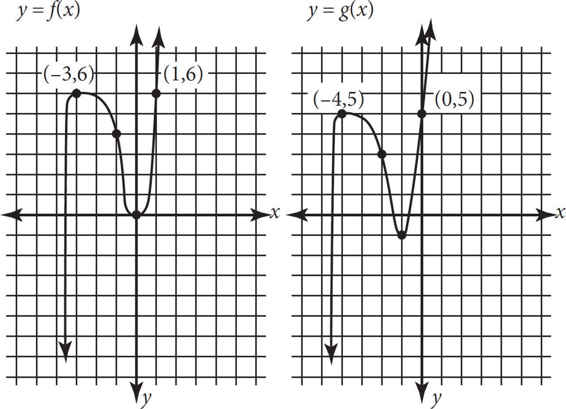# SAT Math Multiple Choice Question 44: Answer and Explanation

### Test Information

Question: 44

4.The figures above show the graphs of the functions f and g. The function f is defined by f(x) = 2x3 + 5x2 - x. The function g is defined by g(x) = f(x - h) - k, where h and k are constants. What is the value of hk ?

• A. -2
• B. -1
• C. 0
• D. 1